# Approaches to Calculating the Cost of Capital

Author: Sophia Tutorial
##### Description:

Differentiate between the types of information derived from the CAPM, discounted cash flow, and BYPRP approaches to calculating the cost of capital.

(more)### Developing Effective Teams

*No strings attached. This college course is 100% free and is worth 1 semester credit.

46 Sophia partners guarantee credit transfer.

299 Institutions have accepted or given pre-approval for credit transfer.

* The American Council on Education's College Credit Recommendation Service (ACE Credit®) has evaluated and recommended college credit for 33 of Sophia’s online courses. Many different colleges and universities consider ACE CREDIT recommendations in determining the applicability to their course and degree programs.

Tutorial

what's covered
In this lesson, you will learn about the types of information derived from the CAPM, discounted cash flow, and BYPRP approaches to calculating the cost of capital. Specifically, this lesson will cover:
1. The Capital Asset Pricing Model
1. Security Market Line
2. Risk and Return
2. Discounted Cash Flow Approach
3. The “Bond Yield Plus Risk Premium” Approach

## 1. The Capital Asset Pricing Model

When considering assets for the diversification of an investment portfolio, investors and financiers use a variety of tools to project the required rate of return and risk of a given investment. All this really means is that investors are on the lookout for ways to minimize risk, maximize returns, and invest intelligently in assets that are well-priced. The key assumption here is that the market will self-correct to adjust each investment option’s expected return to the relative risk of investing.

When measuring the ratio between risk and return on a given investment, the capital asset pricing model (CAPM) can be a useful tool. This model focuses on measuring a given asset’s sensitivity to systematic risk (also referred to as market risk) in relation to the expected return compared to that of a theoretical risk-free asset.

This sounds complicated, but it’s simpler than it seems. All this really means is that the CAPM tries to measure the risk the market will offer the asset compared to the risk-free rate, and make sure the expected return will offset that risk. To understand this concept, there are a few variables that are useful to identify up front:

• E(Ri) is an expected return on security
• E(Rm) is an expected return on market portfolio M
• β is a non-diversifiable or systematic risk
• Rm is a market rate of return
• Rf is a risk-free rate
There are quite a few ways to rearrange the relationship between these variables to derive meaningful information. Like all equations, depending on what you know, you can solve for what you don’t know as well. For a basic CAPM calculation, you would want to solve for the expected return on a security, which looks like this:

formula
Capital Asset Pricing Model (CAPM)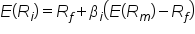term to know

Capital Asset Pricing Model (CAPM)
An equation that assesses the required rate of return on a given investment based upon its risk relative to a theoretical risk-free asset.
1a. Security Market Line
The Security Market Line (SML) is the graphical representation of the CAPM, thus is found using the same equation. The SML shows the x-axis representing the risk (beta), and the y-axis representing the expected return. It graphs the relationship between beta (β) and expected return (i.e., it shows expected return as a function of β). The y-intercept of the SML is equal to the risk-free interest rate, while the slope is equal to the market risk premium (the market’s rate of return minus the risk-free rate). The slope also represents the risk-return tradeoff at a given time. The SML is applicable to any asset.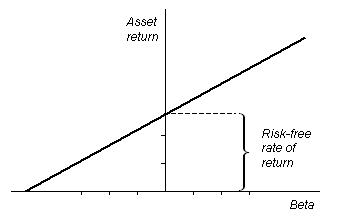Individual assets that are correctly priced are plotted on the SML. In the ideal world of CAPM, all assets are correctly priced and thus lie on the SML. In real market scenarios, we are able to use the SML graph to determine if an asset being considered for a portfolio offers a reasonable expected return for the risk. If an asset is priced at a point above the SML, it is undervalued, since for a given amount of risk, it yields a higher return. Conversely, an asset priced below the SML is overvalued, since for a given amount of risk, it yields a lower return.

Below is the Security Market Line for the Dow Jones Industrial Average over a 3-year period, with the x-axis representing beta and the y-axis representing expected return.big idea
Another way to think about real market applications of the SML would be in terms of buying and selling securities. If an asset is priced above the SML, and thus is undervalued, it should be bought. If an asset is priced below the SML, and thus is overvalued, it should be sold.

term to know

Security Market Line (SML)
The representation of the capital asset pricing model. It displays the expected rate of return of an individual security as a function of systematic, non-diversifiable risk (its beta).
1b. Risk and Return
A final application of the CAPM variables in relation to one another is in deriving a ratio that illustrates the relationship between risk and return. Through rearranging the variables in the CAPM formula, we can see this relationship: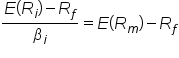On the left side of the equation below, you have an assessment of the overall risk relative to a risk-free asset. On the right side, you have the overall return (similarly relative to a risk-free asset).

Investors can utilize the CAPM equation and its various implications to assess a variety of market investment opportunities to diversify a portfolio and identify undervalued assets.

## 2. Discounted Cash Flow Approach

The discounted cash flow approach is a valuation method investors and organizations can use to assess the net present value (NPV) of an asset, process, product, or the overall organization. NPV analyses using the discounted cash flow approach are widely used across various industries to decide which projects to invest in. They are also used as valuation devices for investors when looking at company performance, using past performance to project future performance.

The discounted cash flow formula focuses on determining the relative time value of money of each projected cash flow (i.e., monthly, quarterly, annually, etc.), bringing each forecast of future value into present value terms. Just as in an NPV analysis, the ultimate end product will be the value of future profits (or losses) in today’s terms. It is calculated as follows:

formula

Discounted Cash Flow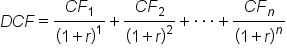In situations of multiple cash flows over multiple periods of time, it is necessary to create a summation that can incorporate variance in both variables, such as:

formula

Discounted Present Value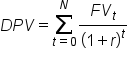When considering these formulas, you’ll need to understand your inputs. The inputs for a discounted cash flow analysis are:
• DPV: The discounted present value of the future cash flow (FV), or FV adjusted to compensate for the units of time in the future it will be received.
• FV: The nominal value of a cash flow amount in a future period (i.e., the amount of the cash flow prior to taking time value of money into account).
• r: The interest rate or discount rate, which reflects two important pieces of information: the opportunity cost of foregoing other investments as well as the intrinsic risk of not receiving the projected cash flow.
• n: The time (in a given unit of time) before the future cash flow occurs. This is usually done annually, but can be done monthly or quarterly.
hint
Keep in mind that r must be adjusted according to the time period!

Like any projection, the most important thing to keep in mind as either investor or strategist is uncertainty. The primary purpose of a future cash flow analysis is to balance expectations to consider existing and future resources to make the optimal decision (from a profit perspective). As a result, determining ‘r’ (required rate of return due to opportunity cost and risk) is absolutely critical to the success of these calculations.

## 3. The “Bond Yield Plus Risk Premium” Approach

The bond yield plus risk premium (BYPRP) approach is another method we can use to determine the value of an asset – specifically, a company’s publicly traded equity. BYPRP allows us to estimate the required return on an equity by adding the equity’s risk premium to the yield to maturity on the company’s long-term debt.

The equation below states that the required return on an equity equals the yield of the company’s long-term debt plus the equity’s risk premium.

formula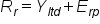Simply put, the yield on a bond is the rate of return received from the investment. In the BYPRP approach, we use a bond’s yield to maturity, which is the discount rate at which the sum of all future cash flows from the bond (coupon payments and principal payments) are equal to the price of the bond. This is also referred to as the internal rate of return (IRR).

The hypothetical graph below shows yield to maturities (or internal rates of return) for corresponding present values.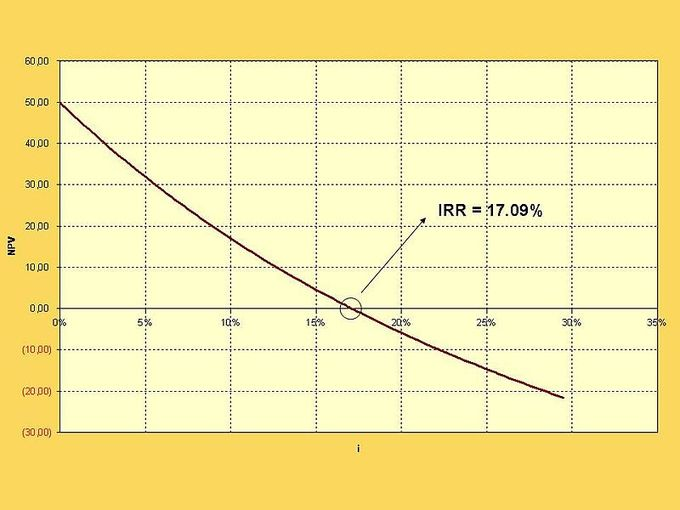The equity risk premium is essentially the return that stocks are expected to receive in excess of the risk-free interest rate. The normal historical equity risk premium for all equities has been just over 6%. In general, an equity’s risk premium will be between 5% and 7%.

Common methods for estimating the equity risk premium include:

• The Fed Model (forward operating earnings yield [earnings] minus the 10-year U.S. Treasury Bond yield)
• The dividend yield plus projected earnings growth, minus the 10-year Treasury yield
• The historical stock returns minus the 10-year Treasury yield
Estimating the value of an equity using the bond yield plus risk premium approach has its drawbacks. We can only utilize the BYPRP approach if the entity has publicly traded debt, and it does not produce as accurate an estimate as the capital asset pricing model or discounted cash flow analysis.

Moreover, equity risk premium estimates can be highly inaccurate, while also varying wildly depending on which model is used. It can be very difficult to get an accurate estimate of the risk premium on an equity, having a duration of roughly 50 years, using a risk-free rate of such short duration as a 10-year Treasury bond.

summary
In this lesson, you learned three approaches to calculating the cost of capital. These include the capital asset pricing model (CAPM), which measures an asset’s sensitivity to market risk in relation to its expected return, compared to that of a theoretical risk-free asset. The Security Market Line and the relationship between risk and return can be derived from the CAPM, which allows investors to assess a variety of market investment opportunities.

You also learned about the discounted cash flow approach to calculating the cost of capital, which can be used to assess net present value (NPV), and the “bond yield plus risk premium” (BYPRP) approach, which can be used to determine the value of a company’s publicly traded equity.

Best of luck in your learning!

Source: THIS CONTENT HAS BEEN ADAPTED FROM LUMEN LEARNING'S “Approaches to Calculating the Cost of Capital” TUTORIAL.

Terms to Know
Capital Asset Pricing Model (CAPM)

An equation that assesses the required rate of return on a given investment based upon its risk relative to a theoretical risk-free asset.

Security Market Line (SML)

The representation of the capital asset pricing model. It displays the expected rate of return of an individual security as a function of systematic, non-diversifiable risk (its beta).

Formulas to KnowCapital Asset Pricing Model (CAPM)Discounted Cash FlowDiscounted Present ValueRating